# Dinesh

Dinesh ate 3 cookies, each with mass 1.45 g. What was the total mass of the cookies that he ate?

Correct result:

x =  4.35 g

#### Solution: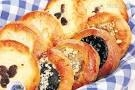We would be pleased if you find an error in the word problem, spelling mistakes, or inaccuracies and send it to us. Thank you!## Next similar math problems:

• Bricks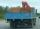Brick has volume 2.2 dm3. How many bricks can drive a truck with a capacity of 23 ton? The density of brick is 1.6 g/cm3.
• Wood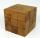Wood cube with edge 11 cm weights 0.753 kg. What weight have 10, 100 and 1000 these cubes?
• The temperatureThe temperature at 1:00 was 10 F. Between 1:00 and 2:00, the temperature dropped 15F. Between 2:00 and 3:00, the temperature rose 3F. What is the temperature at 3:00?
• MO Z6-6-1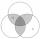Write integers greater than 1 to the blanks in the following figure, so that each darker box was product of the numbers in the neighboring lighter boxes. What number is in the middle box?
• Average priceSixth grade went on a trip to Moravia. Each of the 26 pupils paid CZK 320 and the school paid a total of CZK 3,510. What was the average price of a trip per student?
• Cast brass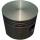Brass cast weight was 3.84 kg, it had turned into piston weight 3.491 kg. How many grams of brass was turned off?
• JagdeepJagdeep had a strip of paper measuring 1.8 m. He cut it into 4 equal strips. How long is each shorter strip of paper?
• NotebooksLiza a store owner buys 560 notebooks. He sold 3/8 of the notebook then she adds the stocks of notebook of 1/4 of the number of notebooks she bought. What is the total number of notebook she bought?
• Three co-owners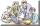The three co-owners of building company have earnings from a contract portioned in the ratio of 3:6:7. Each of them received the amount in whole USD. One of them on contract earned 86450 USD. What was the total earnings this order?
• Pie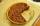Vili ate three pieces of pie . If it piece is 1/8 how much pie did he eat?
• Cake fractions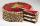Thomas ate 1/3 of cake, Bohouš of the rest of the cake ate 2/5. What fraction of cake left over for others?
• Double 5Peter was thinking of a number. Peter doubles it and gets an answer of 8.6. What was the original number?
• AngieAngie poured 4 cartons of milk, each with 2.4 litres, into a mixing bowl. How many litres of milk were there altogether?
• Cement blocks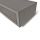Each cement block weighs 7.9 kilograms. How much do 6 blocks weigh in total?
• CupcakesIn a bowl was some cupcakes. Janka ate one third and Danka ate one quarter of cupcakes. a) How many of cookies ate together? b) How many cookies remain in a bowl? Write the results as a decimal number and in notepad also as a fraction.Mom bake cookies. Rolo took 2/9 of all cookies, Michal 3/9. How many cookies ate Rolo if Michal had 9.Suriani had 3.05 m of ribbon. She used up 0.76 m of it. What is the length of ribbon that she has left?